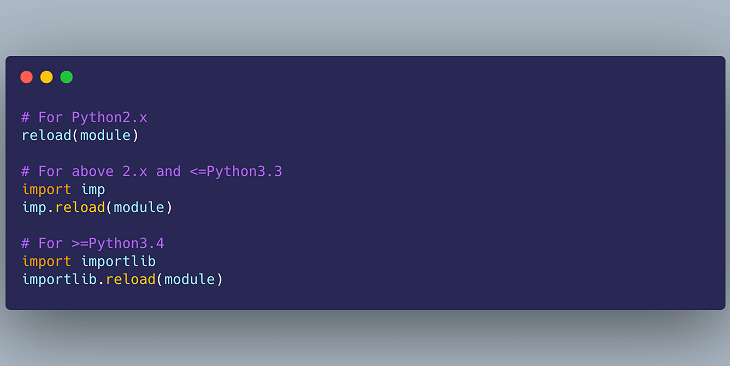# How to Import Python Modules by String Name

Typically, developers import modules in python script by mentioning their name literally. Sometimes you may need to import python modules using string variables that contain their name. In this article, we will learn how to import python modules by string name.

## How to Import Python Modules by String Name

If you are using python <2.7, then you can use __import__ command to import modules using string variables that contain their names.

Let us say you have the following list of strings where each string is a module name, as string.

`moduleNames = ['sys', 'os', 're', 'unittest'] `

If you want to import only a single module using __import__ you can do so using the __import__ function directly. Here is an example to import only unittest module.

`__import__('unittest', globals=globals())`

Here is the command to easily import all the modules mentioned in the above list, using __import__.

`modules = map(__import__, moduleNames)`

Alternatively, and also for python >2.7 you can use importlib module to import modules by their name strings. Here is an example to import module os.path.

`module = importlib.import_module('os.path')`

In this article, we have learnt how to import python modules using their name string.